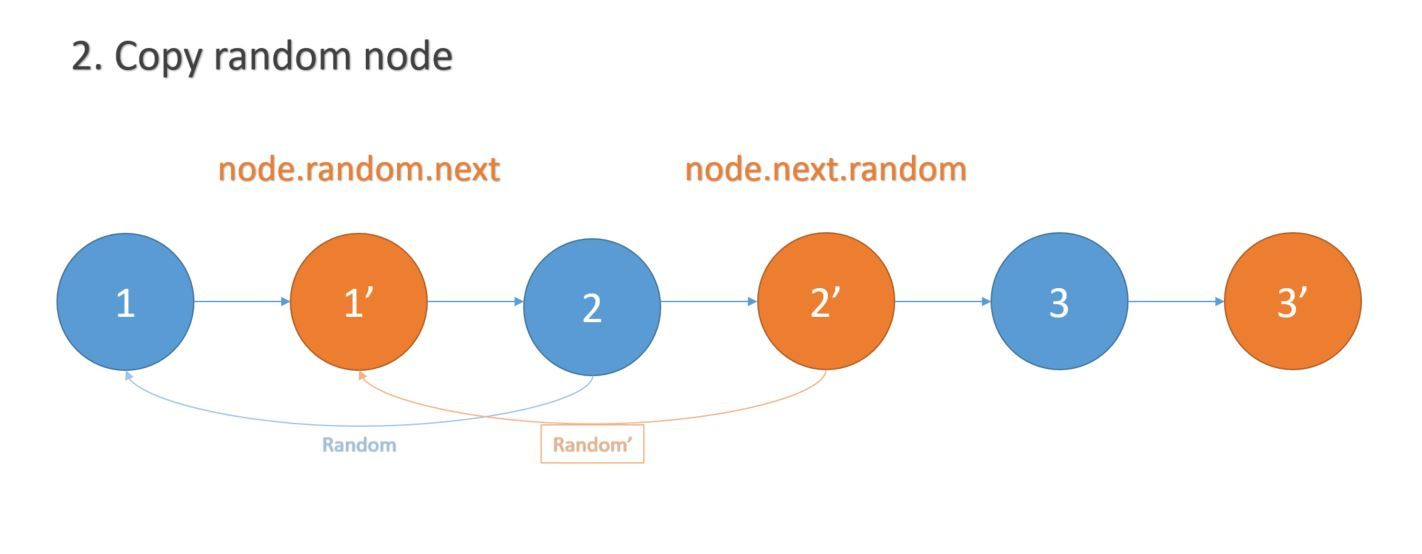### Given a Linked List with random pointers, can you do a deep copy of the list?

The idea is to use a `HashMap` to store the nodes. The key will be the current node and the value will be our new node. If the current node has random node, then we set the random node for our new node as well. Let's see the solution:

``````    public Node copyRandomList(Node head) {

}

Map<Node, Node> map = new HashMap<>();
Node dummy = new Node(0);
Node pre = dummy;
Node newNode = null;

} else{
}
pre.next = newNode;

} else{
}
}
pre = newNode;
}
return dummy.next;
}
``````

Using hash map will allocate `O(n)` space, but can we do it without allocating new space?

The second solution (without hash map) works like this:

1. transform list `1->2->3-4` into `1->1'->2->2'->3->3'->4->4'`
2. copy random node, ex: `1ʳᵃⁿᵈ->5` to `1ʳᵃⁿᵈ'->5'`
3. modify list to return the new copied list only

Here's the solution:

``````    public Node copyRandomList(Node head) {
}

// 1. copy node and add in between current and next node
while(node != null){
Node newNode = new Node(node.val);
newNode.random = node.random;
newNode.next = node.next;
node.next = newNode;
node = node.next.next;
}

// 2. copy random node
while(node != null){
if(node.random != null){
node.next.random = node.random.next;
}
node = node.next.next;
}

Node dummy = new Node(0);
Node prev = dummy;

// 3. split the list into original and copied list
while(node != null){
Node newNode = node.next;
node.next = newNode.next;
prev.next = newNode;
prev = newNode;
node = node.next;
}

return dummy.next;
}
``````

Here's visual graph for understanding:Note | If your newly allocated space is not your input or output, then you are not allocating new extra space.